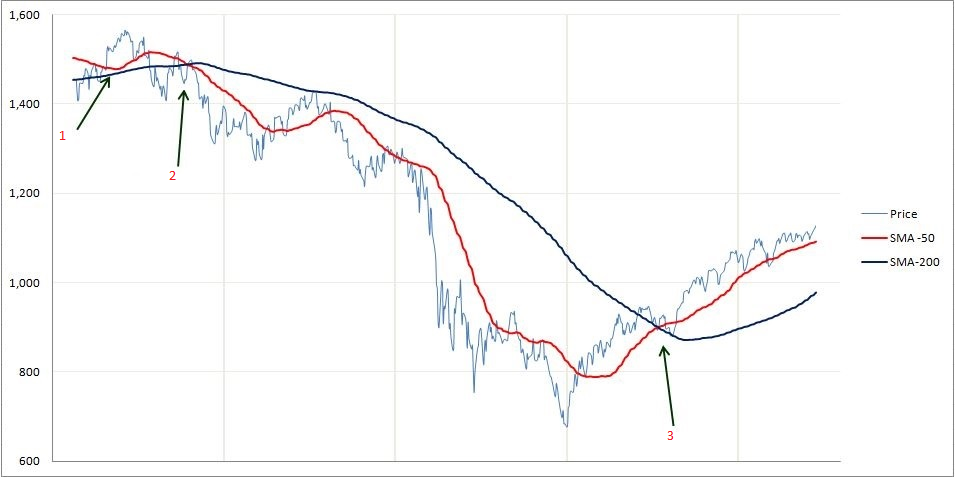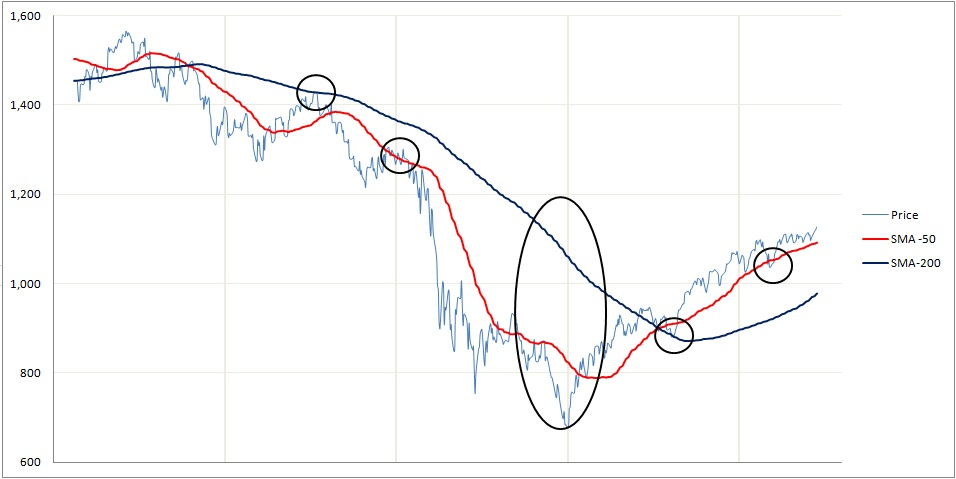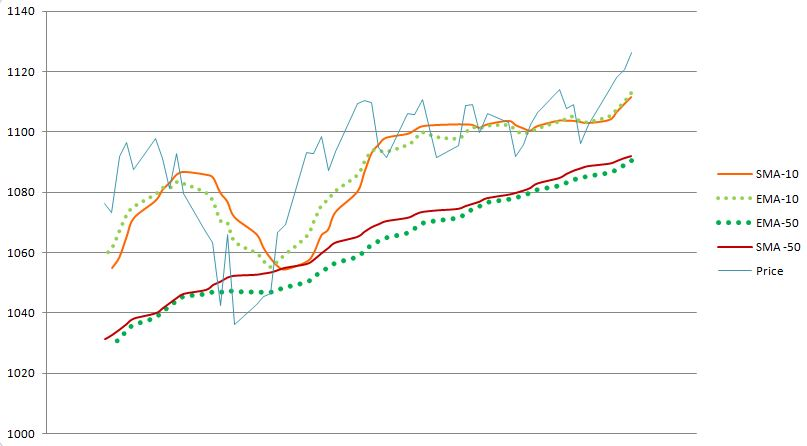# Moving Averages

## Moving Averages

Moving Averages are one of the most popular and important technical analysis tools. The ease of use and simple calculation make it a great tool to get information quickly. They also provide the basics for more advanced technical analysis tools like MACD and Bollinger Bands and can be useful for removing some of the “noise” from daily fluctuations in the market. In simple terms, the moving average is an average that compares the previous period over time. There are two types of moving averages: the Simple Moving Average (SMA) and the Exponential Moving Average (EMA) which puts more weight on the latest date. We will explore the differences more in depth later on.

## Moving Average Technical Analysis

Moving Averages are lagging indicators and give an indication of the strength of a trend rather than predict movement in the asset or market. They are also useful for identifying support and resistance lines and can be used to look at a market reversal. As we will see below, the longer the period used for the SMA, the stronger the trend, hence a 200 day SMA provides a stronger indication of trend than a 10 day SMA.

Moving Averages give a lot of information at crossings between shorter and longer Moving Averages, the distance between the price and the moving average and provide support and resistance lines as well.

Below is a graph with a 50 day and 200 day SMA.The first thing to point out is that the 50 day SMA follows the price much more closely than the 200 day SMA. Hence the longer the period of the SMA, the smoother and more static the line is. As an analogy the 10-day SMA is like a sports car that can turn quick and easily whereas the 200-day SMA is like a large truck that cannot change or turn quickly.

Take note of the points where price and SMA’s or EMA’s cross each other. If we start with the leftmost arrow (1), we can see that the price crosses the 50 day and 200 day multiple times. This is not a strong indication of trend, as the price can frequently give “false” warnings in day to day trading.

A much stronger indication is when a shorter period SMA crosses a longer period SMA. As we can see at the second arrow (2). The 50 day SMA crosses the 200 day SMA from the top (downward) which is a strong indication of a bear market.

On the other hand, the third arrow (3) has the 50 day SMA crossing the 200 day SMA from the bottom (upward) giving a strong indication of a bull market.Another aspect we can see from moving averages is the support and resistance lines. Support lines are when the moving average is below the price and resistance lines are when the moving average is above the price. As we can see from the multiple small circles, the price rarely goes above or below the moving averages for long and even less frequently when it is a longer period. The other thing to notice is the large oval. The greater the distance between a moving average and the underlying price, the more we can expect a reversal of the current trend as we can see above.

!!! Note: These indicators can be useful to determine resistance, support, some reversals and trend strength. However, as you can see from the top left in the graph, this is not always the case. The 50 day SMA came very close to crossing the 200 day average but would not have indicated a very strong trend!

## SMA vs EMA

As we saw earlier the EMA gives more weight to the current price which we will see in more detail in the calculation section.You can see slight discrepancies above between the EMA and SMA. With a price that has more volatility however, you will see greater differences. We can also see that there the EMA tracks some big changes more closely, especially for the EMA that is 10 days as opposed to 50 days.

## Moving Average Calculations

The Simple Moving Average calculation is far more straight forward than the Exponential Moving Average Calculation.

SMA calculation:

N Day SMA: Is the sum of N previous closing prices over N where N is the number of Days considered.

For example: 20 Day SMA is the sum of the closing prices of the 19 previous days(includes today’s closing) over 20.

If we have a 5 day SMA with prices as follows:

 Jan 1st 12.5 Jan 2nd 13.7 Jan 3rd 14.5 Jan 4th 13.9 Jan 5th 16.8

Then the 5-Day SMA on the 5th of January is: (12.5+13.7+14.5+13.9+16.8)/5 = 14.28

!!! You always need the number of days data before you can calculate that day’s Average! So if you want the 200 day SMA for a particular day you need 200 days of data before that!

Mathematical Formula:

(Σ closing price from i-N to i) / N where i is the current day and N is the time period.

EMA calculation:

The EMA is a more complex calculation, though, as we have seen, just as easy to interpret.

EMA = (current Price * 2/(1+N)) + (Previous Day’s EMA * (1-2/(1+N))

The expression 2/(1+N) is called the weighting multiplier and will be higher for smaller periods of EMA. This means that more weight will be given to the last day’s price.

SMA VS EMA

 Price SMA-5 EMA-5 19 17 17 20 18 18 50 24.8 28.67 22 25.8 26.44 23 26.8 25.3

From the table above we can see from the red number in the table, the EMA is much higher than the SMA. This is because the EMA will more closely follow the last price change.